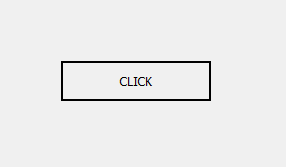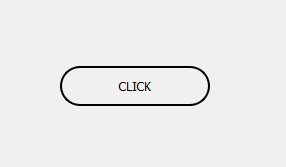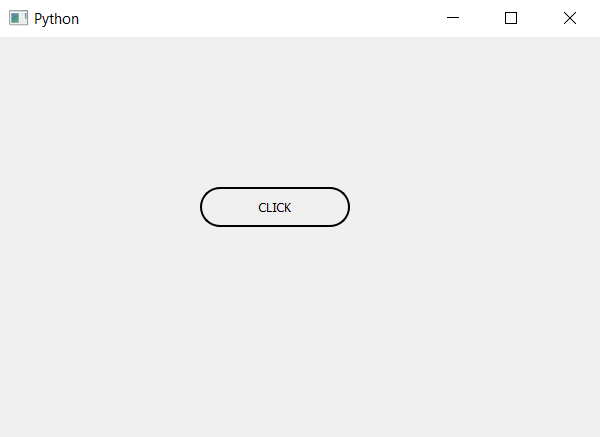# PyQt5 | How to create capsule shaped push button ?

• Last Updated : 22 Apr, 2020

Capsule shape is basically shape which consist of one rectangle and two semi circle is connected to both the ends, in this tutorial we will see how to create these shaped button.

Below is normal Push button vs capsule shaped push button.In order to create capsule shape button we have to do the following steps :

1. Create a button.
2. Resize the button to make it rectangle.
3. Set the radius of button using style sheet to half of height.

Note : If the button will not be rectangle it will become circle.

Code :

 `# importing libraries``from` `PyQt5.QtWidgets ``import` `*` `from` `PyQt5.QtGui ``import` `*` `from` `PyQt5.QtCore ``import` `*` `import` `sys`` ` ` ` `class` `Window(QMainWindow):``    ``def` `__init__(``self``):``        ``super``().__init__()`` ` `        ``# setting title``        ``self``.setWindowTitle(``"Python "``)`` ` `        ``# setting geometry``        ``self``.setGeometry(``100``, ``100``, ``600``, ``400``)`` ` `        ``# calling method``        ``self``.UiComponents()`` ` `        ``# showing all the widgets``        ``self``.show()`` ` `    ``# method for widgets``    ``def` `UiComponents(``self``):`` ` `        ``# creating a push button``        ``button ``=` `QPushButton(``"CLICK"``, ``self``)`` ` `        ``# setting geometry of button``        ``# rectangular shape i.e width > height``        ``button.setGeometry(``200``, ``150``, ``150``, ``40``)`` ` `        ``# adding action to a button``        ``button.clicked.connect(``self``.clickme)`` ` `        ``# setting border and radius``        ``# radius = half of height``        ``button.setStyleSheet("border : ``2px` `solid black; ``                              ``border``-``radius : ``20px``;")`` ` ` ` `    ``# action method``    ``def` `clickme(``self``):`` ` `        ``# printing pressed``        ``print``(``"pressed"``)`` ` `# create pyqt5 app``App ``=` `QApplication(sys.argv)`` ` `# create the instance of our Window``window ``=` `Window()`` ` `# start the app``sys.exit(App.``exec``())`

Output :My Personal Notes arrow_drop_up